## Practice and Learn

 1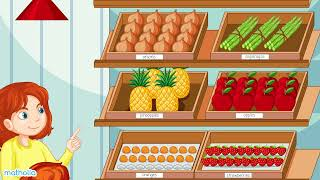Introduction to Multiplication – Skip Counting Video 2Repeated Addition Sheet 3Repeated Addition Word Problems Sheet 4Multiplying by 2 Sheet 5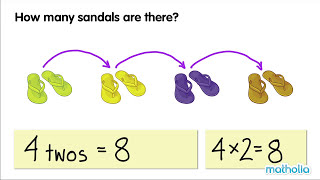Multiplying by 2 Video 6Multiplying by 3 Sheet 7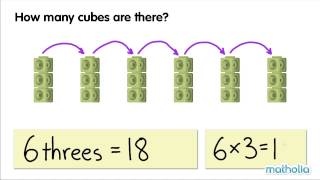Multiplying by 3 Video 8Multiplying by 4 Sheet 9Multiplying by 5 Sheet 10Multiplying by 10 Sheet 11Multiplying by 6 Sheet 12Multiplying by 7 Sheet 13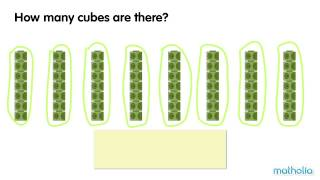Multiplying by 7 Video 14Multiplying by 8 Sheet 15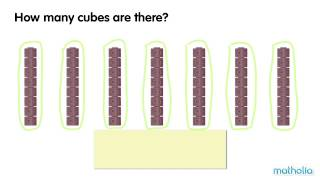Multiplying by 8 Video 16Multiplying by 9 Sheet 17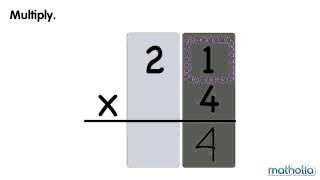Multiplication Without Regrouping Video 18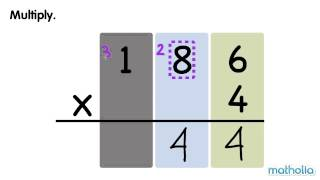Multiplication With Regrouping Video 19Multiplication Word Problems Sheet